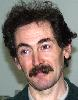Electron. J. Diff. Eqns., Monograph 04 (2003), 85 pages.

### Homogeneous Boltzmann equation in quantum relativistic kinetic theory Miguel Escobedo, Stephane Mischler, & Manuel A. Valle

Abstract:
We consider some mathematical questions about Boltzmann equations for quantum particles, relativistic or non relativistic. Relevant particular cases such as Bose, Bose-Fermi, and photon-electron gases are studied. We also consider some simplifications such as the isotropy of the distribution functions and the asymptotic limits (systems where one of the species is at equilibrium). This gives rise to interesting mathematical questions from a physical point of view. New results are presented about the existence and long time behaviour of the solutions to some of these problems.

Submitted November 29, 2002. Published Janaury 20, 2003.
Math Subject Classifications: 82B40, 82C40, 83-02.
Key Words: Boltzmann equation, relativistic particles, entropy maximization, Bose distribution, Fermi distribution, Compton scattering, Kompaneets equation.

Show me the PDF file (572K), TEX file, and other files for this article.Miguel Escobedo Departamento de Matematicas, Universidad del Pais Vasco Apartado 644 Bilbao E 48080, Spain e-mail: mtpesmam@lg.ehu.es Stephane Mischler DMA, Ecole Normale Superieure Paris Cedex 05 F 75230, France e-mail: Mischler@math.uvsq.fr Manuel A. Valle Departamento de Fisica Teorica e Historia de la Ciencia Universidad del Pais Vasco Apartado 644 Bilbao E48080, Spain email: wtpvabam@lg.ehu.es